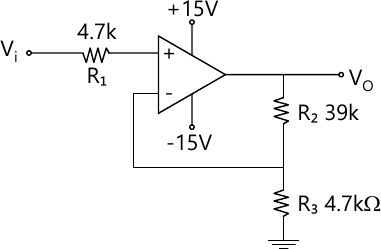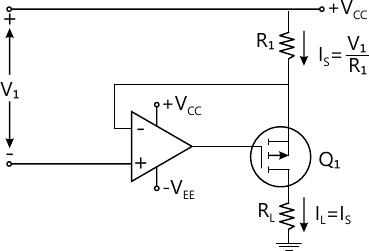MORE IN Linear Integrated Circuits
VTU Electronics and Communication Engineering (Semester 4)
Linear Integrated Circuits
December 2015
Total marks: --
Total time: --
INSTRUCTIONS
(1) Assume appropriate data and state your reasons
(2) Marks are given to the right of every question
(3) Draw neat diagrams wherever necessary

1 (a) With a neat circuit diagram, explain the basic op-amp circuit.
6 M
1 (b) The non-inverting amplifier uses μA 741 op-amp with R1=R3=2.2K and R2=220 K. Determine maximum possible output offset voltage due to:
input offset voltage of 5 mV
ii) input bias current of IB (max)=500 ηA
iii) Input offset current of Ii (OS) = 200 ηA
iv) resistance tolerance of ± 10%.
10 M
1 (c) Obtain the expression for output voltage for the two input inverting summing amplifier circuit.
4 M

2 (a) Draw a neat circuit diagram of a capacitor coupled voltage follower and explain its operation with necessary design steps.
8 M
2 (b) Designed a high impedance capacitor-coupled non-inverting amplifier to have a low cutoff frequency of 200 Hz. The input and output voltages are to be 16 mV and 4V respectively and minimum load resistance is 10 KΩ. Select R2=1 MΩ and C1=0.1 μF.
6 M
2 (c) Explain how the upper cutoff frequency can be set for inverting amplifier with the help of neat circuit diagram and also explain design steps.
6 M

3 (a) Define loop gain, loop phase shift, pole frequency and phase margin.
4 M
3 (b) Explain miller effect compensation.
6 M
3 (c) For the circuit shown in Fig. Q3(c), calculate:
i) Full power bandwidth of 1 V peak input and op-amp slew rate of 250 V/μs
ii) Maximum peak output voltage obtain for input signal of 100 Khz and with slew rate of 0.5 V/μs.4 M
3 (d) List the precautions to be observed for op-amp circuit stability.
6 M

4 (a) Design the current source circuit shown in Fig. Q4(a) to produce a 100mA output to a 40 Ω load. Use ± 12V supply and an LM 108 op-amp.6 M
4 (b) Sketch the circuit of a current amplifier with floating load. Explain circuit operation and derive an equation for current gain.
6 M
4 (c) What are the advantages of precision rectifier over ordinary rectifier? Explain the working of a full wave precision rectifier.
8 M

5 (a) With relevant diagram, explain the operation of negative clamper circuit using op-amp.
6 M
5 (b) Design a triangular waveform generator to produce a ±2V, 1 KHz output. Use a ±15V supply. Also calculate the minimum op-amp slew rate.
8 M
5 (c) Explain the working of phase shift oscillator using op-amp.
6 M

6 (a) With relevant diagram, explain basic inverting and non-inverting comparator circuit with Vref=0V.
6 M
6 (b) With a neat circuit diagram, explain the operation of inverting Schmitt trigger circuit and discuss the design procedure.
10 M
6 (c) Using 741 op-amp, design the first-order active low-pass filter to have a cutoff frequency of 1.2 Khz.
4 M

7 (a) Briefly explain the standard representing of 78XX series 3-terminal IC regulators and enumerate the characteristics of this type of regulators.
8 M
7 (b) With the help of neat diagram, explain the operation of adjustable regulator using fixed 3-terminal regulator.
6 M
7 (c) Explain the operation of basic high voltage regulator using IC 723.
6 M

8 (a) Explain the operation of a mono-stable multivibrator using 555 IC timers.
6 M
8 (b) Explain the operation of phase-locked loop (PPL) with the help of neat block schematic diagram.
8 M
8 (c) What output voltage would be produced by DAC whose output range is 0 to 10 V and whose input binary number is
i) 10 (2 bit DAC)
ii) 0 1 1 0 (4 bit DAC)
iii) 1 0 1 1 1 1 0 0 (for 8 bit DAC).
6 M

More question papers from Linear Integrated Circuits